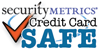item# 2125-1550

# 30" Brass Neck Chain 2.4mm diameter with standard bead clasp

SIZE
30" long ( metric: 762mm )
THICKNESS
3/32" diameter ( metric: 2.4mm ) No.3
QUANTITY
each
MATERIAL
COLOR
Brass
QUANTITY DISCOUNT PRICING

1 to 9 = \$3.99 / each

10 to 24 = \$1.66 / each

25 to 49 = \$0.94 / each

50 to 99 = \$0.78 / each

100 to 249 = \$0.59 / each

250 to 499 = \$0.47 / each

500 to 999 = \$0.46 / each

1000 to 2499 = \$0.42 / each

2500+ = \$0.39 / each

Quantity: